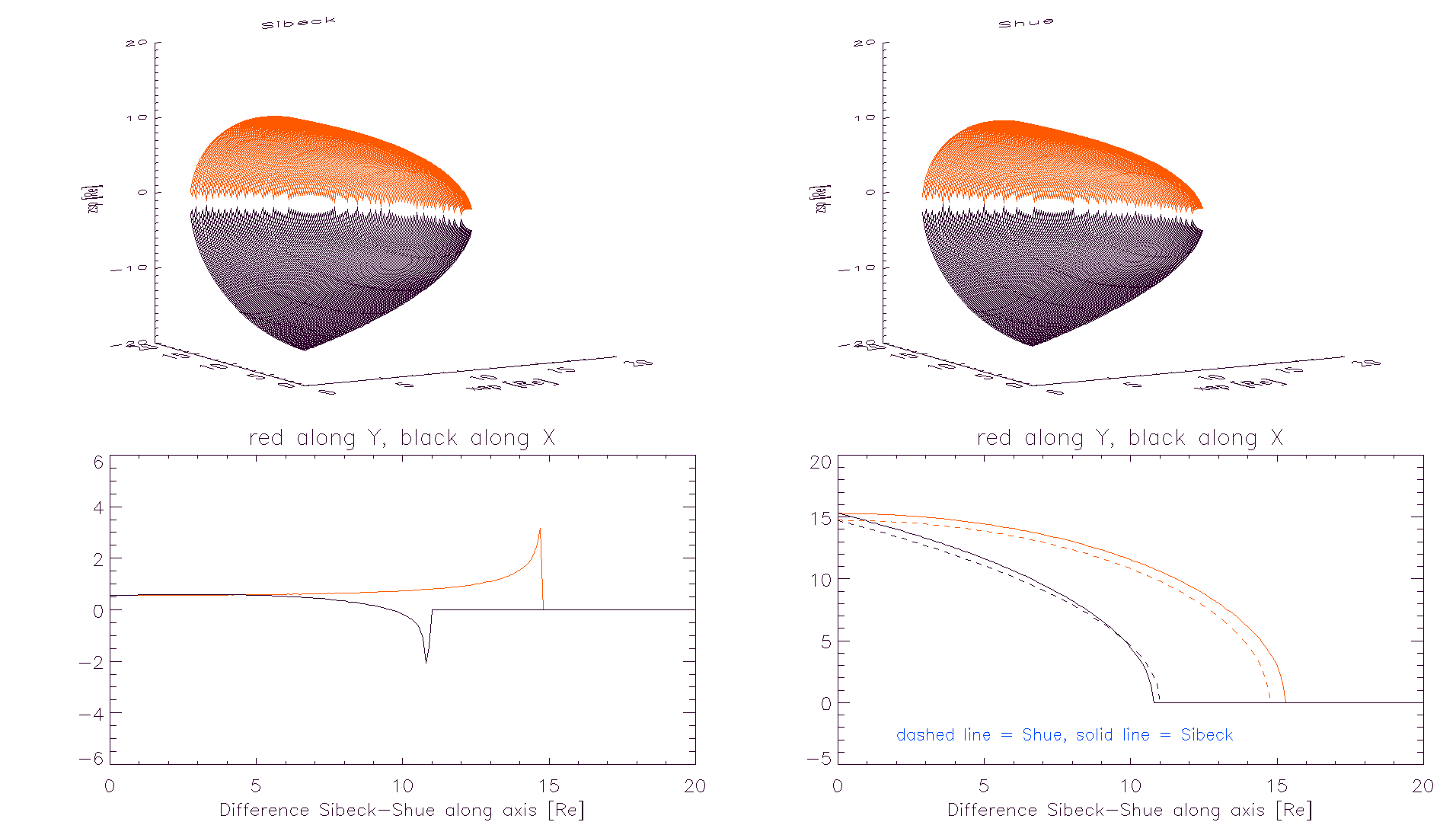One of the difference between the T96 and the TS05 models is the external boundary, i.e. the magnetopause model. As stated before, in the T96 External Field model for the first time a solar wind controlled magnetopause was introduced, namely the Sibeck~\cite{sibeck} model whose size depends from solar wind ram pressure $$P_{dyn}$$, one of the main T96 model parameter, together with the Interplanetary Magnetic Field components $$B_{Y}^{IMF}$$ and $$B_{Z}^{IMF}$$ and $$Dst$$ index. The shape of the magnetopause is described by a function that is similar to an ellipsoid, but the dayside is slightly oblate Solar wind dynamic pressure variations produce self-similar magnetopause motion on time scales of one hour or longer, so the magnetopause moves inward when the solar wind dynamic pressure increases and outward when it decreases. The new magnetopause introduced in the TS05 model is due to Shue. In this model a new functional has been estimated to evaluate the solar wind pressure effect on the magnetopause shape and size, and both the shape and the response to the solar wind have changed with respect to Sibeck ones.